# Moment of Inertia and Oscillations

NOTE

This manual describes the laboratory experiment used during the 1996 - 1997 academic year. Significant changes have been made since then, and the manual used during the current academic year is in NOT available yet on the WEB. Hardcopies can be purchased at the bookstore.

# Moment of Inertia and Oscillations

## Purpose

To measure the moment of inertia of three different objects about a specified rotational axis and to verify the parallel axis theorem.

## Prelab Homework

The prelab homework must be done at home and handed to the lab TA before you start the lab. Read the instructions for this lab.

### Questions

The period of oscillation of a mass M on a spring of mass m is,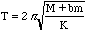(4.1)

where K is the spring constant. Calculate the following for small elongations of the spring, and for small values of m/M, b=1/3.

1) Write down the kinetic energy for an infinitesimal element dm of the spring. Write dm in terms of m, the length of spring L, and an infinitesimal element of the length dx.

[You should get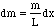].

2) Find an expression for the velocity v(x) of dm at the point x in terms of V, the velocity of the mass M, L the length of spring, and x as it varies from 0 to L.

Hint: The anchored end of the spring is always stationary, so for, say, x = 0, then v = 0. The end where the mass M is attached moves at the same velocity as M, so for x = L, then v = V. Thus, for example at a point halfway between, at x = L/2, then v = V/2. You may assume that the velocity varies linearly from x=0 to x=L.

[You should get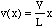.]

3) Find the kinetic energy of the whole spring by integrating over x. Add this to the kinetic energy of M. Compare this kinetic energy to the case when m=0 to see that b=1/3. You also have to assume that the potential energy is, to first order, independent of whether m is finite or not.

[integratewith limits for x=0 to x=L]

4) Review and understand the correspondence between rotational and linear kinematics.

## Part A : Moment of Inertia

Consider a rigid body rotating around an axis. If the angular velocity is [omega], each point in the body will move with linear speed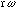, where r is the perpendicular distance of the point from the axis. The total angular momentum L of the rotating body points along the axis and is equal in magnitude to,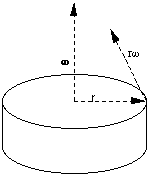Figure 4.1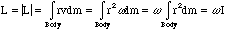(4.2)

where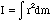is called the moment of inertia of the body about the axis of rotation. In the m.k.s. system of units, the units of I are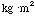. If the axis of rotation is chosen to be through the center of mass of the object, then the moment of inertia about the center of mass axis is call Icm. For example, Icm=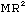for a thin ring of mass M and radius R for the case where the axis is the symmetry axis of the ring. Table 4.1 are examples of Icm for different kinds of objects (e.g. see Ohanian, Chapter 12).

The parallel-axis theorem relates the moment of inertia Icm about an axis through the center of mass to the moment of inertia I about a parallel axis through some other point. The theorem states that,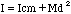(4.3)

 Object Axis Icm Thick Ring (Mass M, radii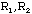) Symmetry Axis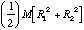Disk (Mass M, radius R) Symmetry Axis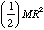Thin Spherical Shell (Mass M, radius R) About a Diameter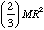Solid Sphere (Mass M, radius R) About a Diameter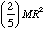Table 4.1

where M is the total mass of the body and d is the distance between the two axis. This implies Icm is always less than I about any other axis.
 Linear Kinematics Rotational Kinematics Velocity = v Angular Velocity =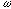Mass = M Moment of Inertia = I Momentum = Mv = P Angular Momentum = L = IKinetic Energy = K =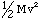Kinetic Energy = K =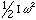Table 4.2

When working with rotational motion for rigid bodies, many of the equations are identical to the equations for linear motion with proper substitutions. The angular velocity is used instead of linear velocity, and the moment of inertia I is used instead of the mass M. Table 4.2 summarizes the correspondence between linear and rotational kinematics for rigid bodies.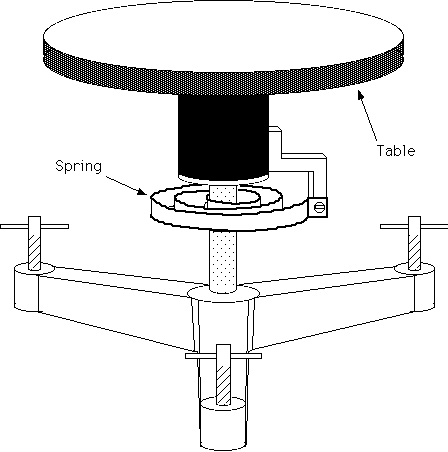Figure 4.2

The apparatus consists of a rotary table on which you can mount the object whose moment of inertia is to be measured. A torsion spring restricts the motion of the table and provides a restoring torque. If the table is rotated by an angle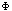, the torque acting on it will be equal to,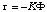(4.4)

where K is a constant which has to be measured. If the sum of the moment of inertia of the table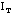and of the object on top I is equal to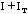, the table will perform a rotary oscillation with the frequency,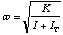(4.5)

which corresponds to a period,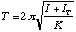(4.6)

Note that there are two unknown parameters of the apparatus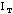and K. To determine these, you need to measure the period of the bare table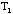, and the period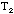resulting when an object of a known moment of inertia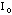is set on the table. Now,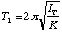(4.7)

and,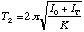(4.8)

So by squaring both equations and then adding we obtain,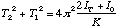(4.9)

and by subtracting,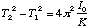(4.10)

This solves for one of the table constants,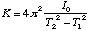(4.11)

The other can be obtained by dividing,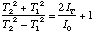(4.12)

or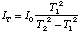(4.13)

By this means the unknown constants of the tableand K can be determined if a body of known moment of inertia is available. Note that the moment of inertia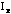of an unknown object can be found using the above equation, i.e.,(4.14)

where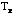is the period with the unknown object on the table.

### Procedure for part A

1) Calculate the moment of inertia of the brass ring from the theoretical formula by measuring the inner and outer radius and the mass by using the formula in Table 4.1.

2) Determine the period of oscillations of the table alone,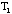.

3) Determine the period of oscillations of the table with the ring,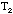.

4) Each measurement should be repeated five times.

5) Use your calculated value for the brass ring as the known moment of inertia and determine K andfor the table using equation (4.13). [In this lab K is somewhere between 0.25 and 1.0 N/m].

6) Measure the periods of oscillation for the disk about five different axis of rotation spaced fairly evenly from the center out to the edge (include one where the axis is centered).

7) Do five trials at each distance. Use these measurements, along with the relation between I and T, to calculate the moment of inertia of the disk at each axis position (using equation 4.14).

8) Weigh the disk and determine its dimensions.

9) Calculate the value of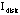with theoretical methods and compare it to the measured value taken when the disk axis is centered on the table. Use the difference as an estimate of the errors.

### Data Analysis for part A

1) With error bars, plot the moment of inertia versus the square of the distance of the center of mass of the disk to the axis of the rotary table.

2) Find the slope and the intercept when you fit your five points to a straight line.

3) Is your value for the slope consistent with the known mass of the brass disk?

4) Does the value of the moment of inertia obtained from the intercept agree with the expected value?

## Part B: Oscillations of a Spiral Spring

It is often assumed that a long spiral spring obeys Hooke's law if it is not stretched too far. If the spring is hung vertically from a fixed support and a mass is attached to its free end, the mass can then oscillate vertically with simple harmonic motion. The period of oscillation depends upon the mass M accelerated and the force constant K of the spring. The period is measured by lifting the weight and letting it go. The time it takes for the weight to return to the start position is defined as the period. If the mass of the spring m is negligible, the period T is,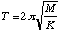(4.15)

However, since the spring also moves, its mass m if not negligible, must enter into the equation for the period. Since the equation must remain dimensionally correct, the mass of the spring can only enter in the following way,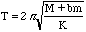(4.16)

where b is a constant number without dimensions.

### Procedure for part B

According to Hooke's Law, the extension of the spring should be proportional to the applied force on the spring. F = - Kx, where F is the force, x is the extension, and K is the force constant of the spring.

1. Determine whether or not the spring obeys Hooke's law by making a plot of the extension of the spring against the attached mass. If it does, measure the slope of the graph and compute the force constant..

2. Find the period for five different attached masses M. (Suggested values: 5, 10, 15, 20, 25 gms)

Be careful not to destroy the spring by over- stretching it. NEVER USE MORE THAN 100g OF WEIGHT! Attach the weights gingerly to the oscillator. Set the oscillator into motion by LIFTING the weight gently (thus compressing the spring) and then releasing.

3. Weigh the spring to determine its mass. There are corrections to be made. Part of the spring is clamped at the top and should be subtracted from the spring mass. The following should be added to the attached mass M: mass of the hook (made of 0.14 m of wire [2.43 gm/m]); mass of the indicator (made of 0.12m [4.6 gm/m]); portion of spring below where the hook is attached.

### Data Analysis for part B.

1) Plot T2 vs. M on a graph. You can measure the period more accurately by measuring the time required for ten complete oscillations and dividing this time by 10 to find the time required for 1 oscillation.

Error Analysis. You may neglect the errors in the masses. Just plot the errors in T on the graph and estimate the errors from the graph as needed.

2) Find the intercept of the graph on the M- axis and calculate the coefficient b assuming the mass of the spring determined above. Compare your experimental value of the constant b with the theoretical value obtained in the prelab question..